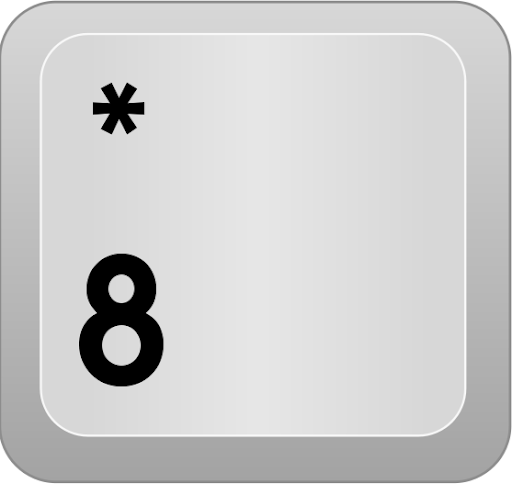### Tips for golfing in JavaScript

•mellamokb

10 years ago

What general tips do you have for golfing in JavaScript? I'm looking for ideas that can be applied to code golf problems in general that are at least somewhat specific to JavaScript (e.g. "remove comments" is not an answer).

Note: Also see Tips for Golfing in ECMAScript 6 and aboveI was actually wondering, is it allowed to put variables in global (saves `var`)? And should JavaScript golf code be a function or output something directly? I honestly think this can make much difference.@primvdb: It is allowed, but you have to be careful because it can cause side-effects if a function is called multiple times and it is manipulating global variables, or if it is a recursive function.This link has a bunch of tips relevant here.

•10 years ago

### Fancy For Loops

you can use the standard for loop in non-standard ways

``for ( a; b; c )``

is essentially equivalent to:

``a;while ( b ){  ...  c;}``

so a good trick is to write your code with a `while` loop, and then split it into the `a,b,c` parts in a `for` loop.

A couple examples I've written:

``for(x=y=n;!z;x--,y++)z=i(x)?x:i(y)?y:0for(a=b=1;b<n;c=a+b,a=b,b=c);``

If you're initializing or resetting multiple values, chain the value to all the variables that need it:

``a=b=1;``

### Implicit Casting

Don't check your types, just use them as they are. `parseInt()` costs `10` characters. If you need to cast out of a string, be creative:

``a='30';b='10';c = a + b; //failurec = parseInt(a) + parseInt(b) //too longc = -(-a-b); //try thesec = ~~a+~~b;c = +a+ +b;c = a- -b;``

### Avoid Semicolons

JavaScript has automatic semi-colon insertion. Use it often and well.

### One-liners

Save on brackets by shoving as much as possible into single lines, or parameters:

``a( realParam1, realParam2, fizz='buzz' )``

### Increment/Decrement operators

``a = a - 1;foo(a);``

and

``foo(a);a = a - 1;``

can easily be rewritten as

``foo(--a);``

and

``foo(a--);``

respectively.

### Use `this` or `self` instead of `window` in global context

self explanatory 2 character savings.

### Use bracket notation for repeat property access

This is definitely a balancing act between property name length and number of accesses. Instead of calling `a.longFunctionName()` with dot notation twice, it's shorter to save the name and call the function via bracket notation:

``a.longFunctionName(b)a.longFunctionName(c)//42``

-vs-

``a[f='longFunctionName'](b)a[f](c)//34``

this is especially effective with functions like `document.getElementById` which can be reduced to `d[e]`.

Note:

With bracket notation, the cost is `6 + name.length` characters the first time. Each subsequent access has a cost of `3` characters.

For dot notation, all accesses cost `name.length + 1` (+1 for the `.`) characters.

Use this method if `6 + name.length + (3 * (accesses - 1)) < accesses * (name.length + 1)`.

len = length of property name

i = minimum accesses to take advantage

``len | i ========1   | ∞ 2   | ∞ 3   | 7 4   | 4 5   | 3 6   | 3 7   | 3 8+  | 2 ``

The number of accesses can also span multiple objects. If you access `.length` 4 or more times on different arrays, you can use the same variable holding the string `'length'`.`c = ~~a-~~b` should be `c = ~~a+~~b`. Also, you can implicitly cast to integer using `|0`, for example `Math.random()*6|0`.It's cheaper to coerce a string to a number with the unary plus operator. If `a` and `b` are strings, you can do `+a+b` to convert to number and add them.Where can I add https://github.com/jed/140bytes/wiki/Byte-saving-techniques ?I swear I'm going to use `d- -b` in my code someday...+a+b doesn't work (at least on mine...) // a="1",b="1",+a+b // gives "11"For "Use Array-Access for repeat function calls" if you're using the function more than twice *on the same object*, what's a bit shorter is to assign the function to a new member like `a.f=a.longfunctionname;a.f(b);a.f(c);a.f(d)`Again, for loops and the stated while loop equivalent will differ in how they handle `continue` keywords.@SuperJedi224, `continue` is far too many characters to be worthwhile. Avoid it.@zzzzBov: In code golf, that is usually true, but that's still a point that's worth mentioning.Instead using `document.getElementById('X').something`, just `X.something` works as wellInstead of `~~a+~~b`, I think you can use `~-a-~b``top` is shorter than `self` or `this`, and it works in local context too